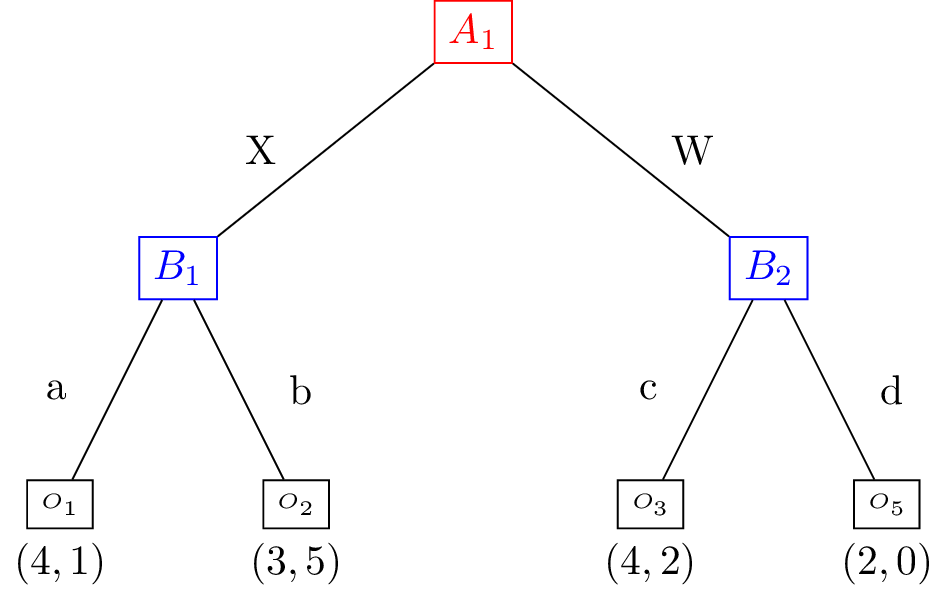# Repeated Games¶

## Motivating example: Repeated Coordination Game¶

Consider the Coordination game but in this instance Alice and Bob repeat their play of this game. In other words, they aim to meet (both making their decision at the same time) and after this first meeting they repeat the process, with full knowledge of the outcome of the first play.

This can be represented pictorially as follows:To show this as an equivalent extensive form game, the tree is the same but we take care to label the vertices correctly:## Definition of a repeated games¶

Given a two player game $$(A,B)\in\mathbb{R}^{{m\times n}^2}$$, referred to as a stage game, a $$T$$-stage repeated game is a game in which players play that stage game for $$T>0$$ repetitions. Players make decisions based on the full history of play over all the repetitions.

Question

For the following values of $$T$$ and the following stage games, how many leaves would the extensive form representation of the repeated game have:

1. $\begin{split}A = \begin{pmatrix}1 & 2 \\ 2 & 3\end{pmatrix} \qquad B = \begin{pmatrix}2 & 3 \\ 1 & -1\end{pmatrix} \qquad T = 2\end{split}$
2. $\begin{split}A = \begin{pmatrix}0 & 1 \\ -1 & 3\end{pmatrix} \qquad B = -A \qquad T = 2\end{split}$
3. $\begin{split}A = \begin{pmatrix}0 & 1 \\ -1 & 3\end{pmatrix} \qquad B = -A \qquad T = 3\end{split}$
4. $\begin{split}A = \begin{pmatrix}0 & 1 & 4\\1 &-1 & 3\end{pmatrix} \qquad B = -A \qquad T = 2\end{split}$

## Strategies in a repeated game¶

A strategy for a player in a repeated game is a mapping from all possible histories of play to a probability distribution over the action set of the stage game.

Question

For the repeated coordination game which of the following are valid strategies, and in the case of valid strategies what is the outcome.

1. For the row player:

\begin{split}\begin{align} (\emptyset, \emptyset) &\to C\\ (S, S) &\to C\\ (S, C) &\to C\\ (C, S) &\to S\\ (C, C) &\to S\\ \end{align}\end{split}

For the column player:

\begin{split}\begin{align} (\emptyset, \emptyset) &\to S\\ (S, S) &\to C\\ (S, C) &\to C\\ (C, S) &\to S\\ (C, C) &\to S\\ \end{align}\end{split}
2. For the row player:

\begin{split}\begin{align} (\emptyset, \emptyset) &\to C\\ (S, S) &\to C\\ (C, S) &\to S\\ (C, C) &\to S\\ \end{align}\end{split}

For the column player:

\begin{split}\begin{align} (\emptyset, \emptyset) &\to S\\ (S, S) &\to C\\ (S, C) &\to C\\ (C, S) &\to S\\ (C, C) &\to S\\ \end{align}\end{split}
3. For the row player:

\begin{split}\begin{align} (\emptyset, \emptyset) &\to C\\ (S, S) &\to C\\ (C, S) &\to S\\ (S, C) &\to S\\ (C, C) &\to S\\ \end{align}\end{split}

For the column player:

\begin{split}\begin{align} (\emptyset, \emptyset) &\to S\\ (S, S) &\to C\\ (S, C) &\to C\\ (C, S) &\to \alpha\\ (C, C) &\to S\\ \end{align}\end{split}
4. For the row player:

\begin{split}\begin{align} (\emptyset, \emptyset) &\to S\\ (S, S) &\to C\\ (C, S) &\to S\\ (S, C) &\to C\\ (C, C) &\to S\\ \end{align}\end{split}

For the column player:

\begin{split}\begin{align} (\emptyset, \emptyset) &\to S\\ (S, S) &\to C\\ (S, C) &\to C\\ (C, S) &\to S\\ (C, C) &\to S\\ \end{align}\end{split}

## Equilibria in repeated games¶

In a repeated game it is possible for players to encode reputation and trust in their strategies.

Consider as an example the following stage game with $$T=2$$:

$\begin{split}A = \begin{pmatrix} 0 & 6 & 1\\ 1 & 7 & 5 \end{pmatrix} \qquad B = \begin{pmatrix} 0 & 3 & 1\\ 1 & 0 & 1 \end{pmatrix}\end{split}$

Through inspection it is possible to verify that the following strategy pair is a Nash equilibrium:

For the row player:

\begin{split}\begin{align} (\emptyset, \emptyset) &\to r_1\\ (r_1, c_1) &\to r_2\\ (r_1, c_2) &\to r_2\\ (r_1, c_3) &\to r_2\\ (r_2, c_1) &\to r_2\\ (r_2, c_2) &\to r_2\\ (r_2, c_3) &\to r_2\\ \end{align}\end{split}

For the column player:

\begin{split}\begin{align} (\emptyset, \emptyset) &\to c_2\\ (r_1, c_1) &\to c_3\\ (r_2, c_1) &\to c_1\\ (r_1, c_2) &\to c_3\\ (r_2, c_2) &\to c_1\\ (r_1, c_3) &\to c_3\\ (r_2, c_3) &\to c_1\\ \end{align}\end{split}

This pair of strategies correspond to the following scenario:

The row player plays $$r_1$$ and the column player plays $$c_2$$ in the first state. The row player plays $$r_2$$ and the column player plays $$c_3$$ in the second stage.

Note that if the row player deviates and plays $$r_2$$ in the first stage then the column player will play $$c_1$$.

If both players play these strategies their utilities are: $$(11, 4)$$ which is better for both players then the utilities at any sequence of pure stage Nash equilibria. But is this a Nash equilibrium? To find out we investigate if either player has an incentive to deviate.

1. If the row player deviates, they would only be rational to do so in the first stage, if they did they would gain 1 in that stage but lose 4 in the second stage. Thus they have no incentive to deviate.

2. If the column player deviates, they would only do so in the first stage and gain no utility.

Thus this strategy pair is a Nash equilibrium and evidences how a reputation can be built and cooperation can emerge from complex dynamics.

## Using Nashpy¶

Repeated games are a particularly compact way of representing a given subset of Extensive Form Games. Thus, it is possible to study them as an equivalent normal form game. See Obtain a repeated game for guidance of how to use Nashpy to generate a normal form game by repeating a stage game.